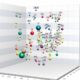# T-LAB 1016 October 2021

### T-LAB 10.2

#### was released on September 24 2023

A new tool, named Co-occurrence Toolkit, added.

This tool, which can be used for a variety of tasks, offers a set of techniques for building and analysing word co-occurrence matrices with up to 5,000 columns.

The matrices to be built can be both symmetric and asymmetric, and they can represent the co-occurrences of the words either within the whole corpus or within a of it.

N.B.: In the case of word co-occurrences, the difference between symmetric and asymmetric matrices is that symmetric matrices assume that the order of words does not matter (i.e., they are represented as undirected graphs where the values in a row and a column are the same), while asymmetric matrices take into account the direction of co-occurrence and, for this reason, are represented as directed graph where the values in a row (i.e., successor) and a column (i.e., predecessor) are not necessarily the same.

Whichever tool you are using, the way to export tables and graphs is very simple (see picture below).

Below is a short description of some of the new analysis options (click here for the complete documentation).

The TOPIC ANALYSIS of the word co-occurrence matrix uses the same algorithm of the T-LAB Modeling of Emerging Themes, tool (i.e., Latent Dirichlet Allocation and the Gibbs Sampling); however, in this case, both the indexes of the matrix (i.e., the ‘i’ and the ‘j’) refer to the same words and the values correspond to their co-occurrences. As can be verified, the results of this approach are quite interesting and consistent.

The RELEVANT WORDS - SVD provides a relevance score for each word, which is computed by summing the square of its first 3 dimensions (i.e., the eigenvectors), each one multiplied by its corresponding singular value, and then by computing the square root of that sum.

This means that the words with the higher scores are the farthest from the point of origin, which is the point where the horizontal axis (x-axis) and the vertical axis (y-axis) intersect. And, for this reason, they are the words that most contribute to organizing semantic polarizations, which can also have emotional connotations.

The SEMANTIC DIVERSITY of each word (i.e., its ability to have links with many other words) is measured by means of the entropy index.

N.B.: The average entropy of the word co-occurrence matrix can be used to quantify the ‘complexity’ of a text, since more complex texts (i.e., texts in which many words cooccur with a variety of other words) tend to have higher entropy than simpler texts (i.e., texts in which many words cooccur with only a few other words and – for that reason – are more predictable).

The ‘local’ CLUSTERING COEFFICIENT is a measure of the degree to which nodes in a graph tend to cluster together and to pair up with each other (i.e., something like ‘The friend of my friend is my friend.’). In other words, the clustering coefficient of a node (i.e., word) quantifies how close its neighbours (i.e., other words) are to being a tightly connected subgroup (i.e., a clique). It is computed as the proportion of the ‘actual’ connections among its neighbours compared with the number of all its ‘possible’ connections. Its maximum value is ‘1’, and the average clustering coefficient of all nodes it is also known as ‘transitivity’ of the network.

N.B. When a network has a large clustering coefficient and a small average path length it can be considered a ‘small world’ (see Wikipedia)

T-LAB 10.1 was releases on December 16 2022.

Here is a short list of the most significant improvements made in this version of the software.

1 – When using the Co-Word Analysis tool, a new interactive dendrogram is available which allows the user to explore the relationships between up 3,000 (three thousand) key-words (see illustrations below).

2 – Now every network graph (force directed graph) available in the T-LAB tools allows the user to highlight the links of each target word on mouse over (see pictures below).

3 – Additionally, both when using the Co-Word Analysis tool and when using the Sequence Analysis tool, a new interactive graph allows the user to dynamically explore the relationship between key-words and the groups to which they belong (see below).

4 – In the new version the available options of the Graph Maker tool, which allows the user to explore various relationships between selected keywords, vary according to the analysis that has been performed previously (see below).

This means that - for example - after performing a thematic analysis or a topic analysis, the user can now explore each cluster/topic by using a 3d PCA (Principal Component Analysis).

5 – Additionally, each time the user edits/modifies a keyword list, he is now enabled to compute and export the TF-IDF values of all items included in his list (see pictures below). In this case, the procedure consists of two steps: (a) computation of the TF-IDF values of a N x M matrix, where ‘N’ are context units (either documents or elementary contexts) and ‘M’ are words (i.e. lemmas); (b) computation of the averaged TF-IDF value of every word within the ‘N’ context units.

6 – Finally, the interactive Word Trees option available through the Concordances tool allows the user to visualize the outputs in three different ways. In fact each root word can be placed either on the left, on the right, or in the centre of its occurrence contexts (see pictures below).